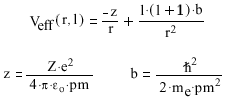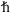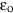﻿

# The Effective Potential for a Hydrogenic Atom

This applet explores how the orbital angular momentum of an electron and the nuclear charge affect the effective potential energy of the electron in a hydrogenic atom. The effective potential energy is given byWhere c is fundamental charge (1.602177 x 10-19 C), me is the mass of an electron (9.10939 x 10-31 kg), r is radius in pm,is h / 2 (1.05457 x 10-34 J s), where h is Planck's constant,is vacuum permittivity (8.85419 x 10-12 J-1 C2 m-1), pm is 1 x 10-12 m, Z is the atomic number and l is the orbital angular momentum quantum number.

To graph effective potential energy enter the parameters (i.e., Z, l) and hit New Plot. Up to 5 plots can be displayed at one time. The Clear button will remove all plots. To see the parameters for each plot hit the Legend on/off button. The Redraw button will refresh the graph. This is useful when the function domain (i.e., radius ) has been changed. To see the value of each plot at a given point, move your cursor to the desired location then click and hold.

To zoom the y-axis about a given value of Veff move the cursor to the left of the y-axis (cursor will change to hand) at the desired value and click. Each click will zoom the scale by 2x. The Redraw and Clear buttons will reset the scale.